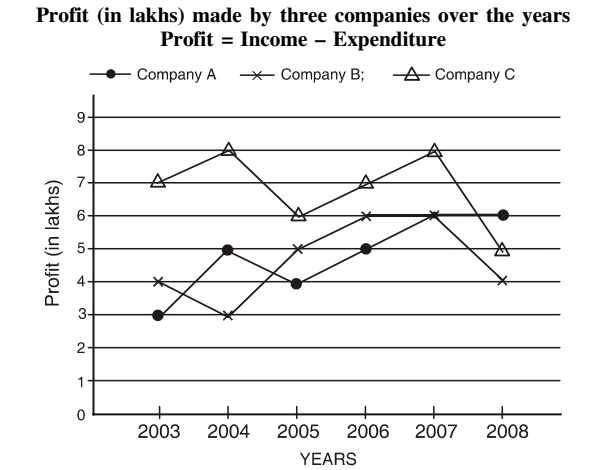## Line chart

#### Data Interpretation

Direction: Study the graph carefully to answer the questions that follow.1. What is the percent increase in profit of company C in the year 2004 from the previous year?

1. Rwquired percentage = 8 - 7/7 x 100 = 14.28% ≈ 14%

##### Correct Option: B

Rwquired percentage = 8 - 7/7 x 100 = 14.28% ≈ 14%

1. Profit made by Company A in the year 2004 was what percent of the total profit made by all the three companies in that year?

1. % Profit made by Company A in the year 2004 = (profit made by A in 2004) x 100/Total profit made by other all 3 companies.

##### Correct Option: C

% Profit made by Company A in the year 2004 = (profit made by A in 2004) x 100/Total profit made by other all 3 companies.
% Profit made by Company A in the year 2004 = 5 x 100/(3 + 5 + 8 ) = 5 x 100/16 = 31.25%

1. If the income of company A in the year 2007 was Rs. 13,54,500. what its expenditure in that year?

1. In 2007, income of Company A = Rs. 13,54,300 and their profit = Rs. 6,00,000

##### Correct Option: B

In 2007, income of Company A = Rs. 13,54,300 and their profit = Rs. 6,00,000
Since, their Expenditure = 13,54,300 - 6,00,000 = Rs. 7,54,300

1. If the expenditure of company B in the year 2008 was Rs. 22,11, 430, what was its income in that year?

1. Expenditure of Company B in 2008 = Rs. 22,11,430 and their profit = Rs. 4,00,000

##### Correct Option: A

Expenditure of Company B in 2008 = Rs. 22,11,430 and their profit = Rs. 4,00,000
Since, Income = 22,11,430 + 4,00,000 = Rs. 26,11,630

1. What is the approximate average profit made by company A in all the years together?

1. Average profit made by company A in all the years = Rs. (3 + 5 + 4 + 5 + 6 + 6)/6

##### Correct Option: C

Average profit made by company A in all the years = Rs. (3 + 5 + 4 + 5 + 6 + 6)lakhs/6
= Rs. 29 /6 ≈ Rs. 4.83 lakh = Rs. 4,83,000